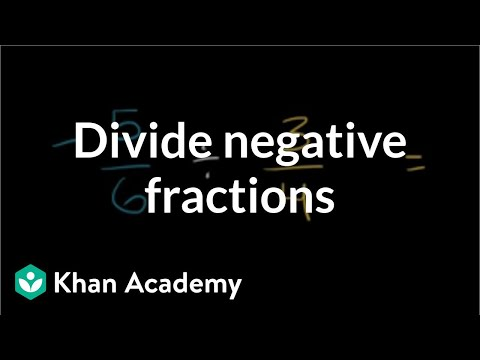# Dividing positive and negative numbers (Full video)

Description: Discover the basics of dividing with negative numbers. Created by Sal Khan. Now that we know a little bit about multiplying positive and negative numbers, Let's think about how how we can divide them. And if both are negative, they'll cancel out and you'll get a positive answer. So if I just said eight (8) divided by two (2), that would be a positive four (4), but since exactly one of these two numbers are negative, this one right over here, the answer is going to be negative.

### Other videos you might be interested in### Dividing negative fractions (Full video)